Courses

# Introduction To Mass Transfer Coefficient Chemical Engineering Notes | EduRev

## Chemical Engineering : Introduction To Mass Transfer Coefficient Chemical Engineering Notes | EduRev

The document Introduction To Mass Transfer Coefficient Chemical Engineering Notes | EduRev is a part of the Chemical Engineering Course Mass Transfer.
All you need of Chemical Engineering at this link: Chemical Engineering

3.1 Introduction to mass transfer coefficient

3.1.1 Concepts of mass transfer coefficients
Movement of the bulk fluid particles in the turbulent condition is not yet thoroughly understood. For gases it is fairly well known as a molecular diffusion since it is described in terms of kinetic theory [1-3]. The rate of mass transfer from the interface to the turbulent zone in the same manner can be useful for molecular diffusion. Thus the term CDAB/Z of Equation (2.13) which is a characteristic of molecular diffusion is replaced by F. For binary solution,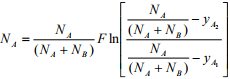(3.1)
The term F is called mass transfer coefficient. The value of F depends on the local nature of the fluid motion. It is a local mass transfer coefficient defined for a particular location on the interface. Its variation depends on the effect of variation in concentrations yA1 and yA2 on the flux. In case of equimolar counter diffusion and transfer of one substance though stagnant another substance, special mass transfer coefficients are generally used which are defined as:

Flux = (a coefficien t) (concentration diffe rence)             (3.2)

How mass transfer rate is calculated when there is bulk motion (turbulent) in the medium? The answer will be addressed in this study. Convective mass transfer is of two types, namely, forced convection mass transfer and free convection mass transfer. The concept of mass transfer coefficient is to develop a simple and practically helpful approach to convective mass transfer problems. Mass transfer rate (WA) is proportional to:
(i) concentration driving force (ΔCA)
(ii) area of the contact between phases (a)
Hence,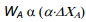(3.3)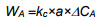(3.4)
where kc is proportionality constant, called mass transfer coefficient. Mass transfer rate, WA can also be expressed in terms of molar flux, NA as: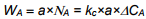(3.5)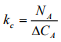(3.6)

3.1.2 Types of mass transfer coefficients
→ Mass transfer occurs in gas/liquid phase
→ Choice of driving force (concentration, partial pressure, mole fraction)
→ Diffusion of “A” through non-diffusing “B”/equimolar counter diffusion of “A” and “B”.

Diffusion of A through non-diffusion B (NB=0)
NA=kG(pA1-pA2)=ky(yA1-yA2)=kc(CA1-CA2) → Gas phase     (3.7)
NA=kx(xA1-xA2)=kL(CA1-CA2) → Liquid phase                       (3.8)
Subscripts (1 and 2) refer two positions in a medium.

For gas phase diffusion we know,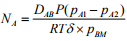(3.9)
where,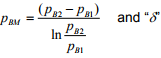is film thickness.

Equating Equation (3.7) and Equation (3.9),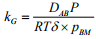(3.10)

Again,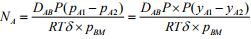(3.11)

Equating Equation (3.7) and Equation (3.11),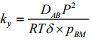(3.12)

Also,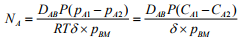(3.13)

Equating Equation (3.7) and Equation (3.13),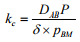(3.14)

For liquid phase diffusion,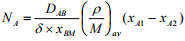(3.15)

where,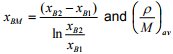is average molar concentration.

Equating Equation (3.8) and Equation (3.15),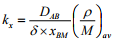(3.16)
Also ,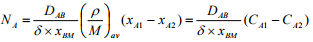(3.17)

Equating Equation (3.8) and Equation (3.17),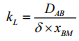(3.18)

Conversions: kc=RT×kG; ky=P×kG;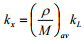Offer running on EduRev: Apply code STAYHOME200 to get INR 200 off on our premium plan EduRev Infinity!

,

,

,

,

,

,

,

,

,

,

,

,

,

,

,

,

,

,

,

,

,

;